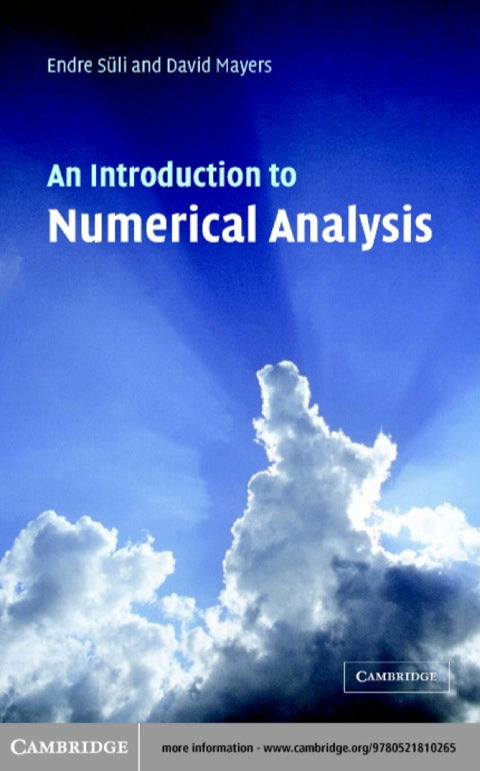• Author(s) Endre Süli; David F. Mayers
• Edition
• Published08282003
• PublisherCambridge University Press
• ISBN9780511074998
Numerical analysis provides the theoretical foundation for the numerical algorithms we rely on to solve a multitude of computational problems in science. Based on a successful course at Oxford University, this book covers a wide range of such problems ranging from the approximation of functions and integrals to the approximate solution of algebraic, transcendental, differential and integral equations. Throughout the book, particular attention is paid to the essential qualities of a numerical algorithm - stability, accuracy, reliability and efficiency. The authors go further than simply providing recipes for solving computational problems. They carefully analyse the reasons why methods might fail to give accurate answers, or why one method might return an answer in seconds while another would take billions of years. This book is ideal as a text for students in the second year of a university mathematics course. It combines practicality regarding applications with consistently high standards of rigour.

# An Introduction to Numerical Analysis

##### Get it instantly

Zookal account needed

\$73.18 \$77.00 Save \$3.82
• Author(s) Endre Süli; David F. Mayers
• Edition
• Published08282003
• PublisherCambridge University Press
• ISBN9780511074998
Numerical analysis provides the theoretical foundation for the numerical algorithms we rely on to solve a multitude of computational problems in science. Based on a successful course at Oxford University, this book covers a wide range of such problems ranging from the approximation of functions and integrals to the approximate solution of algebraic, transcendental, differential and integral equations. Throughout the book, particular attention is paid to the essential qualities of a numerical algorithm - stability, accuracy, reliability and efficiency. The authors go further than simply providing recipes for solving computational problems. They carefully analyse the reasons why methods might fail to give accurate answers, or why one method might return an answer in seconds while another would take billions of years. This book is ideal as a text for students in the second year of a university mathematics course. It combines practicality regarding applications with consistently high standards of rigour.